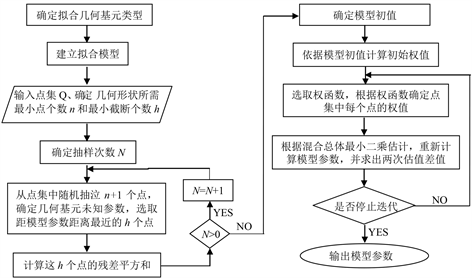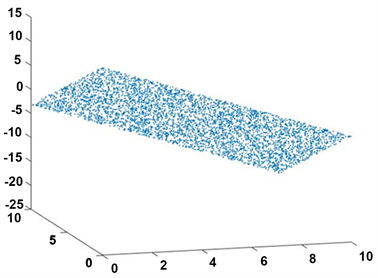﻿ 一种改进的选权迭代算法在点云数据拟合中的应用

一种改进的选权迭代算法在点云数据拟合中的应用Application of an Improved Selecting Weight Iterative Algorithm in Point Cloud Data Fitting

Abstract: To fit the point cloud data with massive outliers, an improved selecting weight iterative algorithm is proposed: the robust initial value is obtained by using the least trimmed squares algorithm, and the number of iterations is judged by the mixed total least squares algorithm; the parameters of the plane fitting and spherical surface fitting are calculated by the method. The result shows: the better parameters are achieved even if the percentage of outliers is high.

1. 引言

2. 改进的选权迭代拟合算法

2.1. 基于最小截断二乘的参数初值估计

1) 从点云数据集(总个数为p)中随机抽取n + 1个点(n为确定拟合模型参数所需的最少点个数)，利用最小二乘法估计参数；

2) 计算点云中所有点在到每次抽样估计模型中的残差，对残差平方进行升序排序，计算前h(h = int(p + n + 1)/2)个残差的平方和作为抽样拟合质量的评价指标。

3) 确定抽样次数N，重复N次上述计算，选取残差平方和最小所对应的模型参数作为选权迭代估计的初值。

2.2. 权函数的选取

${\stackrel{¯}{p}}_{i}=\left\{\begin{array}{ll}{p}_{i}\hfill & |{v}_{i}/{m}_{v}|\le {k}_{0}\text{}\hfill \\ \frac{{k}_{0}}{|{v}_{i}/{m}_{v}|}\left(\frac{{k}_{1}-|{v}_{i}/{m}_{v}|}{{k}_{1}-{k}_{0}}\right){p}_{i}\hfill & {k}_{0}<|{v}_{i}/{m}_{v}|\le {k}_{1}\hfill \\ 0\hfill & {k}_{1}<|{v}_{i}/{m}_{v}|\hfill \end{array}$ (1)

2.3. 选权迭代终止条件

2.4. 执行迭代

3. 点云数据空间平面数据拟合算例分析Figure 1. Flowchart of improved selecting weight iterative algorithmFigure 2. Simulated plane point cloud dataFigure 3. Simulated sphere point cloud dataTable 1. Plane fitting results of different gross errors under selecting weight iterative algorithmTable 2. Plane fitting results of different gross errors under improved selecting weight iterative algorithmTable 3. Sphere fitting results of different gross errors under selecting weight iterative algorithmTable 4. Sphere fitting results of different gross errors under improved selecting weight iterative algorithm

4. 点云数据球面数据拟合算例分析

5. 结束语

 邓念武, 李萌. 实物逆向工程中全站扫描仪精度分析[J]. 测绘通报, 2016(3): 146-147.

 官云兰, 程效军, 施贵刚. 一种稳健的点云数据平面拟合方法[J]. 同济大学学报(自然科学版), 2008, 36(7): 981-984.

 游俊甫. 基于RANSAC的点云数据特征提取[D]: [硕士学位论文]. 抚州: 东华理工大学, 2015.

 李明磊, 李广云, 王力. 点云平面拟合新方法[C]//全国工程测量2012技术研讨交流会论文集. 2012.

 周江文. 抗差最小二乘法[M]. 武汉: 华中理工大学出版社, 1997.

 杨飚, 张曾科, 孙政顺. 基于LTS稳健初值的选权迭代法[J]. 科学技术与工程, 2005(22): 30-33, 37.

 程效军, 李杰. 具有最小截断二乘稳健初值的点云平面拟合算法[J]. 同济大学学报(自然科学版), 2015, 43(9): 1419-1424.

 龚循强, 李志林. 一种利用IGG II方案的稳健混合总体最小二乘方法[J]. 武汉大学学报(信息科学版), 2014, 39(4): 462-466.

Top